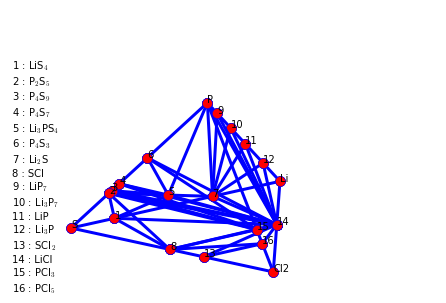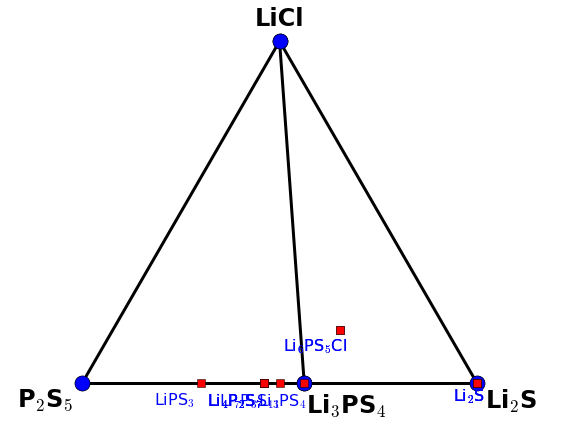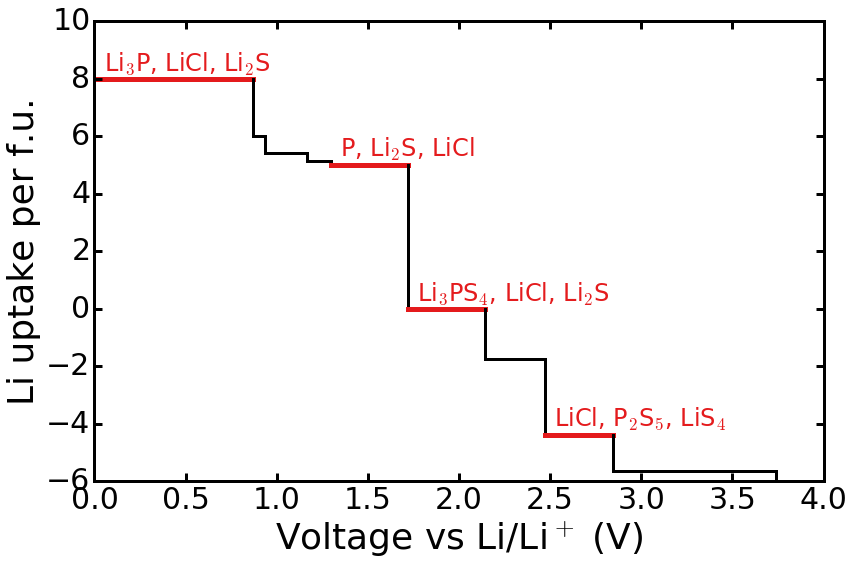Introduction¶

This notebook demonstrates how to perform phase and electrochemical assessments starting from a VASP calculation using Python Materials Genomics (pymatgen) and the Materials Project database (via the Materials API). These notebooks are described in detail in

Deng, Z.; Zhu, Z.; Chu, I.-H.; Ong, S. P. Data-Driven First-Principles Methods for the Study and Design of
Alkali Superionic Conductors. Chem. Mater. 2017, 29 (1), 281–288 DOI: 10.1021/acs.chemmater.6b02648.



If you find these notebooks useful and use the functionality demonstrated, please consider citing the above work.

Let's start by importing some modules and classes that we will be using.

In :
%matplotlib inline

from pymatgen import MPRester, Composition, Element
from pymatgen.io.vasp import Vasprun
from pymatgen.phasediagram.maker import PhaseDiagram, CompoundPhaseDiagram
from pymatgen.phasediagram.analyzer import PDAnalyzer
from pymatgen.phasediagram.plotter import PDPlotter
from pymatgen.entries.computed_entries import ComputedEntry
from pymatgen.entries.compatibility import MaterialsProjectCompatibility
from pymatgen.util.plotting_utils import get_publication_quality_plot
import json
import re
import palettable
import matplotlib as mpl


Preparation¶

We will first read the results from the vasprun.xml output file from our VASP calculations. Only the lowest energy result is used here.

In :
vasprun = Vasprun("aimd_data/vasprun.xml.relax2.gz")
# include structure so proper correction can be applied for oxides and sulfides
entry = vasprun.get_computed_entry(inc_structure=True)


To construct the phase diagram, we need all entries in the Li-P-S-Cl chemical space. We will use the MPRester class to obtain these entries from the Materials Project via the Materials API.

In :
rester = MPRester()
mp_entries = rester.get_entries_in_chemsys(["Li", "P", "S", "Cl"])


In addition to all the MP entries, here we also load the computed entries of O/S substituted Li-P-O tenary compounds.

In :
with open("aimd_data/lpo_entries.json") as f:
lpo_entries = [ComputedEntry.from_dict(d) for d in lpo_data]


Next, we need to combine all the entries and postprocess them using MaterialsProjectCompatibility. This postprocessing step corrects the energies to account for well-known DFT errors, e.g., in the sulfur binding energy.

In :
compatibility = MaterialsProjectCompatibility()
entry = compatibility.process_entry(entry)
entries = compatibility.process_entries([entry] + mp_entries + lpo_entries)


Phase diagram construction¶

The phase diagram can then be constructed using the PhaseDiagram class, and plotted using the PDPlotter class.

In :
pd = PhaseDiagram(entries)
plotter = PDPlotter(pd)
plotter.show()We may observe from the above phase diagram that Li6PS5Cl is not a stable phase (red nodes) in the calculated 0K phase diagram.

The pseudo-ternary Li2S-P2S5-LiCl is constructed using the CompoundPhaseDiagram class.

In :
cpd = CompoundPhaseDiagram(entries,
[Composition("P2S5"), Composition("Li2S"), Composition("LiCl")])
cplotter = PDPlotter(cpd, show_unstable=True)
cplotter.show()Calculating $E_{\rm hull}$ of Li6PS5Cl¶

We may evaluate the $E_{\rm hull}$ of Li6PS5Cl using the PDAnalyzer.

In :
analyzer = PDAnalyzer(pd)
ehull = analyzer.get_e_above_hull(entry)
print("The energy above hull of Li6PS5Cl is %.3f eV/atom." % ehull)

The energy above hull of Li6PS5Cl is 0.021 eV/atom.


Electrochemical Stability¶

The electrochemical stability can be assessed using a similar phase diagram approach, but using the lithium grand potential instead of the internal energy.

First, we need to identify a reference for lithium chemical potential using the bulk Li energy $\mu_{\rm Li}^0$.

In :
li_entries = [e for e in entries if e.composition.reduced_formula == "Li"]
uli0 = min(li_entries, key=lambda e: e.energy_per_atom).energy_per_atom


The PDAnalyzer class provides a quick way to plot the phase diagram at a particular composition (e.g., Li6PS5Cl) as a function of lithium chemical potential called get_element_profile.

In :
el_profile = analyzer.get_element_profile(Element("Li"), entry.composition)
for i, d in enumerate(el_profile):
voltage = -(d["chempot"] - uli0)
print("Voltage: %s V" % voltage)
print(d["reaction"])
print("")

Voltage: -0.0 V
4.000 Li6PS5Cl + 32.000 Li -> 4.000 Li3P + 4.000 LiCl + 20.000 Li2S

Voltage: 0.8693499325 V
4.000 Li6PS5Cl + 24.000 Li -> 4.000 LiP + 20.000 Li2S + 4.000 LiCl

Voltage: 0.934797859375 V
4.000 Li6PS5Cl + 21.714 Li -> 0.571 Li3P7 + 20.000 Li2S + 4.000 LiCl

Voltage: 1.16859139812 V
4.000 Li6PS5Cl + 20.571 Li -> 4.000 LiCl + 20.000 Li2S + 0.571 LiP7

Voltage: 1.29794984292 V
4.000 Li6PS5Cl + 20.000 Li -> 4.000 P + 20.000 Li2S + 4.000 LiCl

Voltage: 1.71892977688 V
4.000 Li6PS5Cl -> 0.000 Li + 4.000 Li3PS4 + 4.000 LiCl + 4.000 Li2S

Voltage: 2.14019005929 V
4.000 Li6PS5Cl -> 7.000 Li + 4.000 Li3PS4 + 4.000 LiCl + 1.000 LiS4

Voltage: 2.47188645738 V
4.000 Li6PS5Cl -> 17.500 Li + 4.000 LiCl + 2.000 P2S5 + 2.500 LiS4

Voltage: 2.846213603 V
4.000 Li6PS5Cl -> 22.500 Li + 4.000 SCl + 2.000 P2S5 + 1.500 LiS4

Voltage: 3.74073532125 V
4.000 Li6PS5Cl -> 24.000 Li + 2.000 P2S5 + 6.000 S + 4.000 SCl



This element profile can be plotted as a Li evolution versus voltage using matplotlib as follows.

In :
# Some matplotlib settings to improve the look of the plot.
mpl.rcParams['axes.linewidth']=3
mpl.rcParams['lines.markeredgewidth']=4
mpl.rcParams['lines.linewidth']=3
mpl.rcParams['lines.markersize']=15
mpl.rcParams['xtick.major.width']=3
mpl.rcParams['xtick.major.size']=8
mpl.rcParams['xtick.minor.width']=3
mpl.rcParams['xtick.minor.size']=4
mpl.rcParams['ytick.major.width']=3
mpl.rcParams['ytick.major.size']=8
mpl.rcParams['ytick.minor.width']=3
mpl.rcParams['ytick.minor.size']=4

# Plot of Li uptake per formula unit (f.u.) of Li6PS5Cl against voltage vs Li/Li+.

colors = palettable.colorbrewer.qualitative.Set1_9.mpl_colors
plt = get_publication_quality_plot(12, 8)

for i, d in enumerate(el_profile):
v = - (d["chempot"] - uli0)
if i != 0:
plt.plot([x2, x2], [y1, d["evolution"] / 4.0], 'k', linewidth=3)
x1 = v
y1 = d["evolution"] / 4.0
if i != len(el_profile) - 1:
x2 = - (el_profile[i + 1]["chempot"] - uli0)
else:
x2 = 5.0

if i in [0, 4, 5, 7]:
products = [re.sub(r"(\d+)", r"$_{\1}$", p.reduced_formula)
for p in d["reaction"].products if p.reduced_formula != "Li"]

plt.annotate(", ".join(products), xy=(v + 0.05, y1 + 0.3),
fontsize=24, color=colors)

plt.plot([x1, x2], [y1, y1], color=colors, linewidth=5)
else:
plt.plot([x1, x2], [y1, y1], 'k', linewidth=3)

plt.xlim((0, 4.0))
plt.ylim((-6, 10))
plt.xlabel("Voltage vs Li/Li$^+$ (V)")
plt.ylabel("Li uptake per f.u.")
plt.tight_layout()